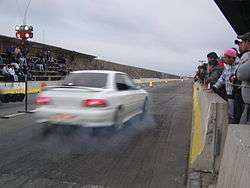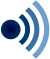# Speed

"Slow" and "Slowness" redirect here. For other uses, see Slow (disambiguation) and Slowness (disambiguation).
SpeedSpeed can be thought of as the rate at which an object covers distance. A fast-moving object has a high speed and covers a relatively large distance in a given amount of time, while a slow-moving object covers a relatively small amount of distance in the same amount of time.
Common symbols
v
SI unit m/s, m s−1

In everyday use and in kinematics, the speed of an object is the magnitude of its velocity (the rate of change of its position); it is thus a scalar quantity. The average speed of an object in an interval of time is the distance travelled by the object divided by the duration of the interval; the instantaneous speed is the limit of the average speed as the duration of the time interval approaches zero.

Speed has the dimensions of distance divided by time. The SI unit of speed is the metre per second, but the most common unit of speed in everyday usage is the kilometre per hour or, in the US and the UK, miles per hour. For air and marine travel the knot is commonly used.

The fastest possible speed at which energy or information can travel, according to special relativity, is the speed of light in a vacuum c = 299792458 metres per second (approximately 1079000000 km/h or 671000000 mph). Matter cannot quite reach the speed of light, as this would require an infinite amount of energy. In relativity physics, the concept of rapidity replaces the classical idea of speed.

## Definition

The Italian physicist Galileo Galilei is credited with being the first to measure speed by considering the distance covered and the time it takes. Galileo defined speed as the distance covered per unit of time. In equation form, this iswhereis speed,is distance, andis time. A cyclist who covers 30 metres in a time of 2 seconds, for example, has a speed of 15 metres per second. Objects in motion often have variations in speed (a car might travel along a street at 50 km/h, slow to 0 km/h, and then reach 30 km/h).

In mathematical terms, the speedis defined as the magnitude of the velocity, that is, the derivative of the positionwith respect to time:Ifis the length of the path travelled until time, the speed equals the time derivative of:In the special case where the velocity is constant (that is, constant speed in a straight line), this can be simplified to. The average speed over a finite time interval is the total distance travelled divided by the time duration.

### Instantaneous speed

Speed at some instant, or assumed constant during a very short period of time, is called instantaneous speed. By looking at a speedometer, one can read the instantaneous speed of a car at any instant. A car travelling at 50 km/h generally goes for less than one hour at a constant speed, but if it did go at that speed for a full hour, it would travel 50 km. If the vehicle continued at that speed for half an hour, it would cover half that distance (25 km). If it continued for only one minute, it would cover about 833 m.

### Average speed

Different from instantaneous speed, average speed is defined as the total distance covered divided by the time interval. For example, if a distance of 80 kilometres is driven in 1 hour, the average speed is 80 kilometres per hour. Likewise, if 320 kilometres are travelled in 4 hours, the average speed is also 80 kilometres per hour. When a distance in kilometres (km) is divided by a time in hours (h), the result is in kilometres per hour (km/h). Average speed does not describe the speed variations that may have taken place during shorter time intervals (as it is the entire distance covered divided by the total time of travel), and so average speed is often quite different from a value of instantaneous speed. If the average speed and the time of travel are known, the distance travelled can be calculated by rearranging the definition toUsing this equation for an average speed of 80 kilometres per hour on a 4-hour trip, the distance covered is found to be 320 kilometres.

Expressed in graphical language, the slope of a tangent line at any point of a distance-time graph is the instantaneous speed at this point, while the slope of a chord line of the same graph is the average speed during the time interval covered by the chord.

### Tangential speed

Linear speed is the distance travelled per unit of time, while tangential speed (or tangential velocity) is the linear speed of something moving along a circular path. A point on the outside edge of a merry-go-round or turntable travels a greater distance in one complete rotation than a point nearer the center. Travelling a greater distance in the same time means a greater speed, and so linear speed is greater on the outer edge of a rotating object than it is closer to the axis. This speed along a circular path is known as tangential speed because the direction of motion is tangent to the circumference of the circle. For circular motion, the terms linear speed and tangential speed are used interchangeably, and both use units of m/s, km/h, and others.

Rotational speed (or angular speed) involves the number of revolutions per unit of time. All parts of a rigid merry-go-round or turntable turn about the axis of rotation in the same amount of time. Thus, all parts share the same rate of rotation, or the same number of rotations or revolutions per unit of time. It is common to express rotational rates in revolutions per minute (RPM) or in terms of the number of "radians" turned in a unit of time. There are little more than 6 radians in a full rotation (2π radians exactly). When a direction is assigned to rotational speed, it is known as rotational velocity or angular velocity. Rotational velocity is a vector whose magnitude is the rotational speed.

Tangential speed and rotational speed are related: the greater the RPMs, the larger the speed in metres per second. Tangential speed is directly proportional to rotational speed at any fixed distance from the axis of rotation. However, tangential speed, unlike rotational speed, depends on radial distance (the distance from the axis). For a platform rotating with a fixed rotational speed, the tangential speed in the centre is zero. Towards the edge of the platform the tangential speed increases proportional to the distance from the axis. In equation form:where v is tangential speed and ω (Greek letter omega) is rotational speed. One moves faster if the rate of rotation increases (a larger value for ω), and one also moves faster if movement farther from the axis occurs (a larger value for r). Move twice as far from the rotational axis at the centre and you move twice as fast. Move out three times as far and you have three times as much tangential speed. In any kind of rotating system, tangential speed depends on how far you are from the axis of rotation.

When proper units are used for tangential speed v, rotational speed ω, and radial distance r, the direct proportion of v to both r and ω becomes the exact equationThus, tangential speed will be directly proportional to r when all parts of a system simultaneously have the same ω, as for a wheel, disk, or rigid wand.

## Units

Units of speed include:

Conversions between common units of speed
m/s km/h mph knot ft/s
1 m/s = 1 3.6 2.236936 1.943844 3.280840
1 km/h = 0.277778 1 0.621371 0.539957 0.911344
1 mph = 0.44704 1.609344 1 0.868976 1.466667
1 knot = 0.514444 1.852 1.150779 1 1.687810
1 ft/s = 0.3048 1.09728 0.681818 0.592484 1

(Values in bold face are exact.)

## Examples of different speeds

Speed m/s ft/s km/h mph Notes
Approximate rate of continental drift 0.00000001 0.00000003 0.00000004 0.00000002 4 cm/year. Varies depending on location.
Speed of a common snail 0.001 0.003 0.004 0.002 1 millimetre per second
A brisk walk 1.7 5.5 6.1 3.8
A typical road cyclist 4.4 14.4 16 10 Varies widely by person, terrain, bicycle, effort, weather
A fast martial arts kick 7.7 25.2 27.7 17.2 Fastest kick recorded at 130 milliseconds from floor to target at 1 meter distance. Average velocity speed across kick duration
Sprint runners 12.2 40 43.92 27 Usain Bolt's 100 metres world record.
Approximate average speed of road cyclists 12.5 41.0 45 28 On flat terrain, will vary
Typical suburban speed limit in most of the world 13.8 45.3 50 30
Taipei 101 observatory elevator 16.7 54.8 60.6 37.6 1010 m/min
Typical rural speed limit 24.6 80.66 88.5 56
British National Speed Limit (single carriageway) 26.8 88 96.56 60
Category 1 hurricane 33 108 119 74 Minimum sustained speed over 1 minute
Speed limit on a French autoroute 36.1 118 130 81
Highest recorded human-powered speed 37.02 121.5 133.2 82.8 Sam Whittingham in a recumbent bicycle
Muzzle velocity of a paintball marker 90 295 320 200
Cruising speed of a Boeing 747-8 passenger jet 255 836 917 570 Mach 0.85 at 35000 ft (10668 m) altitude
The official land speed record 341.1 1119.1 1227.98 763
The speed of sound in dry air at sea-level pressure and 20 °C 343 1125 1235 768 Mach 1 by definition. 20 °C = 293.15 kelvins.
Muzzle velocity of an AK47 assault rifle bullet 710 2330 2600 1600
Official flight airspeed record for jet engined aircraft 980 3215 3530 2194 Lockheed SR-71 Blackbird
Space shuttle on re-entry 7800 25600 28000 17,500
Escape velocity on Earth 11200 36700 40000 25000 11.2 km·s−1
Voyager 1 relative velocity to the Sun in 2013 17000 55800 61200 38000 Fastest heliocentric recession speed of any humanmade object. (11 mi/s)
Average orbital speed of planet Earth around the Sun 29783 97713 107218 66623
The fastest recorded speed of the Helios probes. 70,220 230,381 252,792 157,078 Recognized as the fastest speed achieved by a man-made spacecraft, achieved in solar orbit.
Speed of light in vacuum (symbol c) 299792458 983571056 1079252848 670616629 Exactly 299792458 m/s, by definition of the metre

## Psychology

According to Jean Piaget, the intuition for the notion of speed in humans precedes that of duration, and is based on the notion of outdistancing. Piaget studied this subject inspired by a question asked to him in 1928 by Albert Einstein: "In what order do children acquire the concepts of time and speed?" Children's early concept of speed is based on "overtaking", taking only temporal and spatial orders into consideration, specifically: "A moving object is judged to be more rapid than another when at a given moment the first object is behind and a moment or so later ahead of the other object."

## ReferencesLook up speed or swiftness in Wiktionary, the free dictionary.Wikiquote has quotations related to: Speed
1. Wilson, Edwin Bidwell (1901). Vector analysis: a text-book for the use of students of mathematics and physics, founded upon the lectures of J. Willard Gibbs. p. 125. This is the likely origin of the speed/velocity terminology in vector physics.
2. Hewitt (2006), p. 42
3. Hewitt (2006), p. 131
4. Hewitt (2006), p. 132
5. http://www.kickspeed.com.au/Improve-measure-kicking-speed.html
6. http://www.wisil.recumbents.com/wisil/whpsc2009/results.htm
7. Darling, David. "Fastest Spacecraft". Retrieved August 19, 2013.
8. Jean Piaget, Psychology and Epistemology: Towards a Theory of Knowledge, The Viking Press, pp. 82–83 and pp. 110–112, 1973. SBN 670-00362-x
9. Siegler, Robert S.; Richards, D. Dean (1979). "Development of Time, Speed, and Distance Concepts" (PDF). Developmental Psychology. 15 (3): 288–298. doi:10.1037/0012-1649.15.3.288.
10. Rod Parker-Rees and Jenny William, eds. (2006). Early Years Education: Histories and Traditions, Volume 1. Taylor & Francis. p. 164.